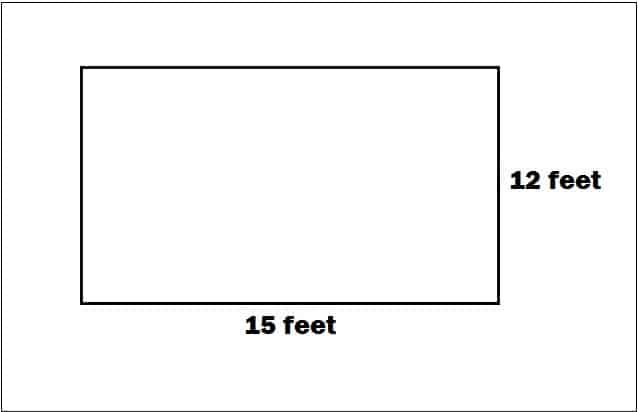# How To Calculate Quantity Of Paint For Building

Contents

## How To Calculate Quantity Of Paint For Building:

Painting is one of the most essential steps to be done after construction of any building/house. Painting is done to protect the surface of the walls as well as to increase appearance.

In this article, I will discuss how to calculate the quantity of paint required in a building.

Before starting the calculation let me tell you one important thing that quantity of paint is calculated in gallon.

There are two types of gallons

US gallon and Uk gallon.

1 US gallon = 3.785 litres

1 Uk gallon = 4.546 litres.

In this calculation we will use US gallon.Let the length of the wall = 15 feet

The height of the wall = 12 feet.

Area of the wall 15 x 12 = 180 sft.

## Thumb Rule For Paint Calculation:

Generally, 1 gallon of paint can cover up to 350 sft area of wet wall and

1 gallon of paint can cover up to 200 sft area of dry wall.

## Paint Quantity Of Wet wall:

Area of wet wall =180 sft

Paint covered area = 300 sft

Quantity of paint = 180/350 = 0.514 litre.

Let the price of 1 gallon paint = Rs 1200.

Price of 0.514 gallon paint = 0.514 x 1200 =  617 Rs.

## Paint Quantity Of Dry Wall:

Area of dry wall = 180 sft.

Pain covered area = 200

Quantity of paint = 180/200 =0.9 gallon.

Price of 0.9 gallon paint = 0.9 x 1200 = 1080 Rs.

Note:

1. If the wall has any openings, subtract the area of openings from total area & then calculate paint quantity.

### 7 thoughts on “How To Calculate Quantity Of Paint For Building”

1. Nice one.Tanks alot.